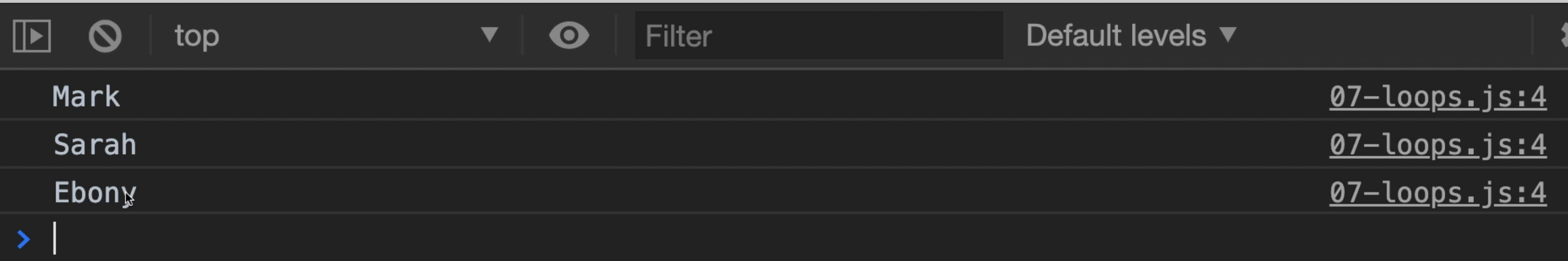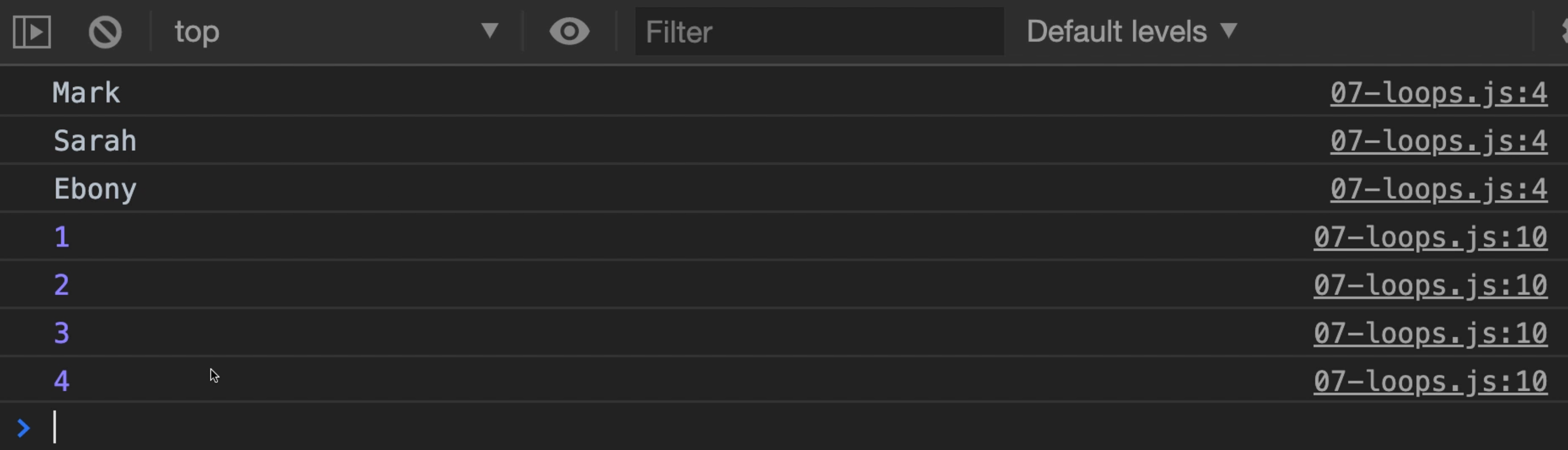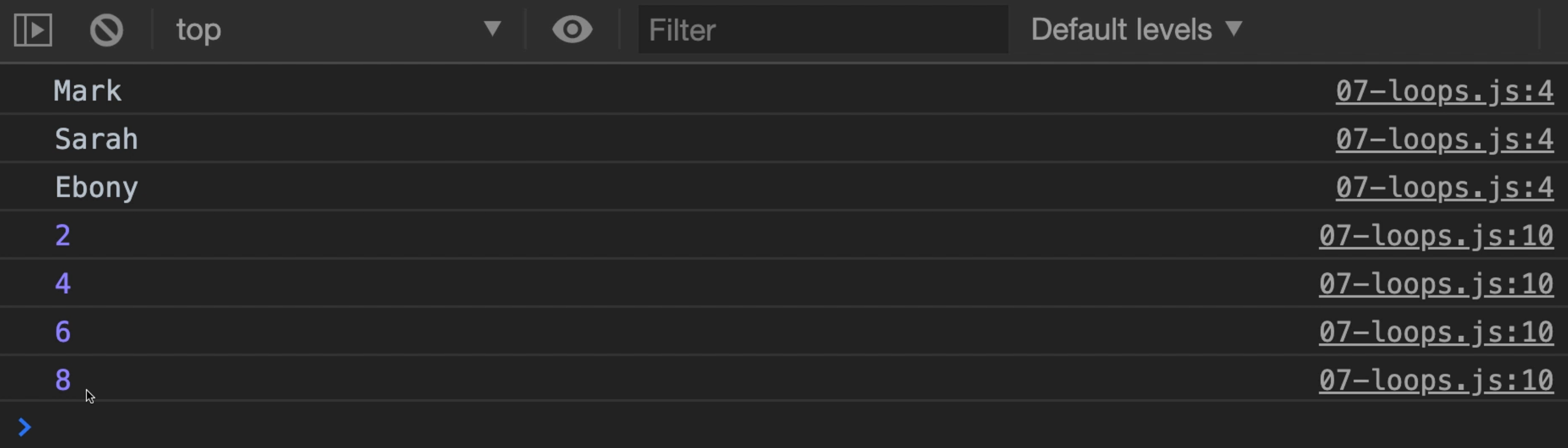Transcripted Summary

There's one more foundational concept that we need to cover in this course. Let's jump into it.

Suppose that I had an array of five names and I asked you to display each name one at a time. You could probably write that code with something like this.

``````console.log(name);
console.log(name);
console.log(name);
console.log(name);
console.log(name);
``````

And you would do this like you see on the screen about five times. This seems reasonable, but here's where things get a little weird.

What if I had 50 names in the array?

You could probably figure out a way to write this code 50 times, but you would probably find yourself making an error somehow.

What if you didn't know how many items were in the array beforehand and you needed to write the code to make this happen?

Wouldn't it be nice if there were a way to repeat a block of code over and over again?

Well, you are in luck because JavaScript supports this concept and it's called loops.

And loops do just what we need.

Loops

Loops allow us to repeat code over and over again.

And there are multiple types of loops. Let's jump into the code and have a look at a few different types. We'll be using file `07-loops.js`.

### # Classic for loop

First up is the modern classic `for` loop.

A `for` loop is absolutely great for repeating a task, a set number of times.

Here are the main parts of the `for`.

#### # Initialization

First, you start with the `for` keyword.

This lets JavaScript know the type of construct we'll be using.

On `line 1`, I used the `let` keyword to declare a variable called `i` and give the initial value of `0`.

``````for (let i = 0;)
``````

#### # Condition

After the initialization, we have what's called the condition.

How many times should I do this loop?

If the condition sounds familiar, well, it's because we've seen it before when working with `if` statements - the same rules apply here.

The most common condition that you'll see when starting out with your programming is `i` less than `array.length`.

You can have other variations here, but this is usually a good place to start.

``````for (let i = 0; i < names.length;)
``````

Once we've defined our condition, the next step is to decide how you want to update your counter variable or how you want to increment it.

#### # Increment

Commonly you will increment or update the value by one, but you run into times where you want to do something a little different.

Maybe you want to increment by a different number or even you may want to go backwards to decrement.

Being able to customize a `for` loop is just another example of being flexible in programming.

``````for (let i = 0; i < names.length; i++)
``````

The `++` means add 1 to `i`.

We'll finish our structure with curly braces `{}`.

Now, anything inside the curly braces will be executed the number of times from `0` - while starting from `0` - while `i` is less than `names.length`.

The only thing that's left to do now is to define the loop body or what's the logic that we want to execute.

Let's have a look at the `for` loop again from our original hypothetical.

So we have our `for` loop, let's add our `names` array.

``````const names = ["Mark", "Sarah", "Ebony"];

for (let i = 0; i < names.length; i++) {

}
``````

Inside, we're going to go over each element of our array, so we'll do it this way. We'll do `console.log`, the `names` variable, our brackets `[]`, then we'll use `i` here.

``````const names = ["Mark", "Sarah", "Ebony"];

for (let i = 0; i < names.length; i++) {
console.log(names[i]); // 0, 1, 2
}
``````

During the life of the `for` loop, I will start at 0, go 1, then 2, because 2 is going to be less than `names.length`, which is 3.

But remember, we're starting at 0.

So we're going to get 1, 2, 3 iterations, and that's going to take us where we want.

And we should see these names on the console using this code.

Let's save it and let's have a look in the browser.Now here's the great thing. We see "Mark", "Sarah" and "Ebony" in order as they appear in the array.

And you see that these all come from the same line in the code, which is `line 4`. This is really incredible.

This is introducing a new level of sophistication to your code, because now you can have code that can be repeated a set number of times, the same way you might see when you're doing your tests.

You might see your test repeated a certain number of times based on some inputs.

Okay, let's look at another example.

What if we have an array of numbers and we wanted to double each of these numbers.

To do this, we need to go through each of the items of the array and do some logic. Okay, let's give it a try.

Let's create our `numbers` array. Then we need our `for` loop.

First, we start with the keyword `for` then our parentheses.

We'll do `let`. We've used `i` already and technically we could use it again.

But for clarity sake, we're going to use the next letter in the alphabet.

Let's start with `j`. We'll set `j` equal to `0` .

Just a quick note about `i` versus `j`.

Why didn't I use `x`? Why didn't I use `a` or some other letter?

Well, this is a very standard practice and programming that you start with `i` and you start using letters after `i`, so you use `i`, then `j`, then `k`, and then you continue through the alphabet.

This is just something that's been around for a very long time. There is no rule that says you have to do it, but this is what we call convention. And this convention actually goes beyond JavaScript. It goes across most, if not all, programming languages.

Next up, we have our condition. Well, we want to say while `j` is less than `numbers.length`, then we'll `console.log` the array `numbers`. And then we'll put in our variable `j` and then finish with a semicolon `;`.

``````const names = ["Mark", "Sarah", "Ebony"];

for (let i = 0; i < names.length; i++) {
console.log(names[i]); // 0, 1, 2
}

const numbers = [1, 2, 3, 4];

for (let j = 0; j < numbers.length; j++) {
console.log(numbers[j]);
}
``````

Let's save it and go to the browser and test this out.Okay, we only output the values, but we didn't double them. Let's fix that.

To double a number, it would really be times two.

So I'm going to say this `*` for times, and then the number `2`, and that gives us our formula.

``````const names = ["Mark", "Sarah", "Ebony"];

for (let i = 0; i < names.length; i++) {
console.log(names[i]); // 0, 1, 2
}

const numbers = [1, 2, 3, 4];

for (let j = 0; j < numbers.length; j++) {
console.log(numbers[j] * 2);
}
``````

Let's go back and refresh.Now you see `2`, `4`, `6`, `8`. Who do we appreciate? Mark, yeah!

Okay, so we've just had our introduction to `for` loops.

For Loops

For loops allow us to repeat code a set number of times. And that time is usually based on the condition.

Typically, we start at `0` and then we iterate a certain number of times, but those rules are flexible.

Sometimes you might want to start at a higher number or a different number, or even a calculated number, but that's where `for` loops reign supreme because they are extremely flexible.

And in this case, we use `j++`, which is the same as saying `j = j+1`.

Now `for` loops are just one of multiple types of loops that we have access to.

To learn about the other two, why don't you head over to the next video? Stay tuned and stay awesome. We'll see you in the next one.

## Resources

Quiz

The quiz for this chapter can be found in section 6.2